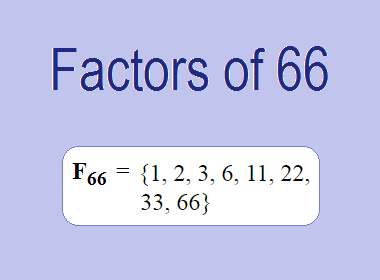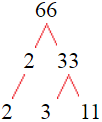# Factors of 66The factors of 66 are 1, 2, 3, 6, 11, 22, 33, and 66 i.e. F66 = {1, 2, 3, 6, 11, 22, 33, 66}. The factors of 66 are all the numbers that can divide 66 without leaving a remainder.

We can check if these numbers are factors of 66 by dividing 66 by each of them. If the result is a whole number, then the number is a factor of 66. Let's do this for each of the numbers listed above:

·        1 is a factor of 66 because 66 divided by 1 is 66.

·        2 is a factor of 66 because 66 divided by 2 is 33.

·        3 is a factor of 66 because 66 divided by 3 is 22.

·        6 is a factor of 66 because 66 divided by 6 is 11.

·        11 is a factor of 66 because 66 divided by 11 is 6.

·        22 is a factor of 66 because 66 divided by 22 is 3.

·        33 is a factor of 66 because 66 divided by 33 is 2.

·        66 is a factor of 66 because 66 divided by 66 is 1.

## How to Find Factors of 66?

1 and the number itself are the factors of every number. So, 1 and 66 are two factors of 66. To find the other factors of 66, we can start by dividing 66 by the numbers between 1 and 66. If we divide 66 by 2, we get a remainder of 0. Therefore, 2 is a factor of 66. If we divide 66 by 3, we get a remainder of 0. Therefore, 3 is also a factor of 66.

Next, we can check if 4 is a factor of 66. If we divide 66 by 4, we get a remainder of 2. Therefore, 4 is not a factor of 66. We can continue this process for all the possible factors of 66.

Through this process, we can find that the factors of 66 are 1, 2, 3, 6, 11, 22, 33, and 66. These are the only numbers that can divide 66 without leaving a remainder.

********************

********************

## Properties of the Factors of 66

The factors of 66 have some interesting properties. One of the properties is that the sum of the factors of 66 is equal to 144. We can see this by adding all the factors of 66 together:

1 + 2 + 3 + 6 + 11 + 22 + 33 + 66 = 144

Another property of the factors of 66 is that the prime factors of 66 are 2, 3 and 11 only.

## Applications of the Factors of 66

The factors of 66 have several applications in mathematics. One of the applications is in finding the highest common factor (HCF) of two or more numbers. The HCF is the largest factor that two or more numbers have in common. For example, to find the HCF of 66 and 55, we need to find the factors of both numbers and identify the largest factor they have in common. The factors of 66 are 1, 2, 3, 6, 11, 22, 33, and 66. The factors of 55 are 1, 5, 11, and 55. The largest factor that they have in common is 11. Therefore, the HCF of 66 and 55 is 11.

Another application of the factors of 66 is in prime factorization. Prime factorization is the process of expressing a number as the product of its prime factors. The prime factors of 66 are 2, 3 and 11, since these are the only prime numbers that can divide 66 without leaving a remainder. Therefore, we can express 66 as:

66 = 2 × 3 × 11

We can do prime factorization by division and factor tree method also. Here is the prime factorization of 66 by division method,66 = 2 × 3 × 11

Here is the prime factorization of 66 by the factor tree method,66 = 2 × 3 × 11

## Conclusion

The factors of 66 are the numbers that can divide 66 without leaving a remainder. The factors of 66 are 1, 2, 3, 6, 11, 22, 33, and 66. The factors of 66 have some interesting properties, such as having a sum of 144. The factors of 66 have several applications in mathematics, such as finding the highest common factor and prime factorization.